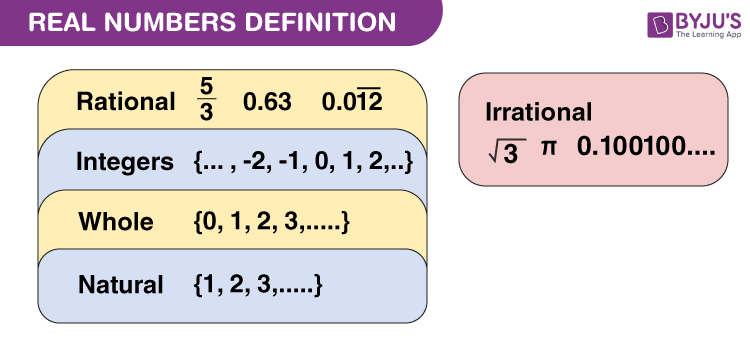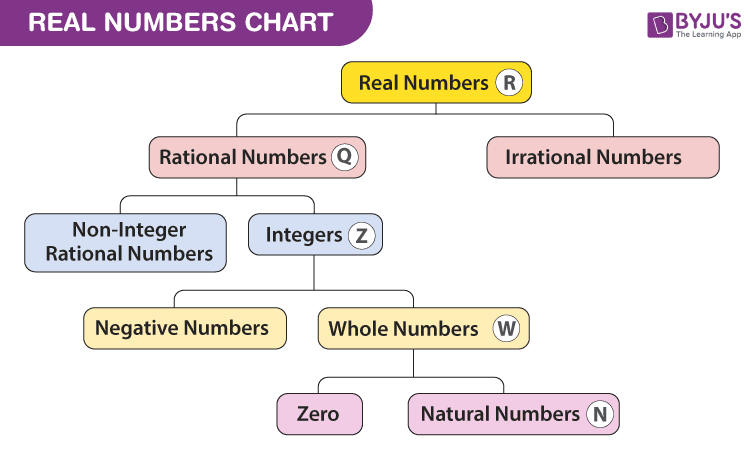Real Numbers

Real Numbers

Real numbers are simply the combination of rational and irrational numbers, in the number system. In general, all the arithmetic operations can be performed on these numbers and they can be represented in the number line, also. At the same time, the imaginary numbers are the un-real numbers, which cannot be expressed in the number line and is commonly used to represent a complex number. The concepts related to real numerals are explained here in detail, along with examples and practice questions. The key concept in the number system is included in this article.

 Examples 23 -12 6.99 5/2 π(3.14)

Real Numbers Definition

Real numbers can be defined as the union of both the rational and irrational numbers. They can be both positive or negative and are denoted by the symbol “R”. All the natural numbers, decimals and fractions come under this category. See the figure, given below, which shows the classification of real numerals.Set of Real Numbers

The set of real numbers consist of different categories, such as natural and whole numbers, integers, rational and irrational numbers. In the table given below, all these numbers are defined with examples.

Category Definition Example
Natural Numbers Contain all counting numbers which start from 1.N = {1,2,3,4,……} All numbers such as 1, 2, 3, 4,5,6,…..…
Whole Numbers Collection of zero and natural number.W = {0,1,2,3,…..} All numbers including 0 such as 0, 1, 2, 3, 4,5,6,…..…
Integers The collective result of whole numbers and negative of all natural numbers. Includes: -infinity (-∞),……..-4, -3, -2, -1, 0, 1, 2, 3, 4, ……+infinity (+∞)
Rational Numbers Numbers that can be written in the form of p/q, where q≠0. Examples of rational numbers are ½, 5/4 and 12/6 etc.
Irrational Numbers All the numbers which are not rational and cannot be written in the form of p/q. Irrational numbers are non-terminating and non-repeating in nature like √2

Real Numbers Chart

The chart for the set of real numerals including all the types are given below:Properties of Real Numbers

There are four main properties which include commutative property, associative property, distributive property and identity property. Consider “m, n and r” are three real numbers. Then the above properties can be described using m, n, and r as shown below:

Commutative Property

If m and n are the numbers, then the general form will be m + n = n + m for addition and m.n = n.m for multiplication.

• Addition: m + n = n + m. For example, 5 + 3 = 3 + 5, 2 + 4 = 4 + 2
• Multiplication: m × n = n × m. For example, 5 × 3 = 3 × 5, 2 × 4 = 4 × 2

Associative Property

If m, n and r are the numbers. The general form will be m + (n + r) = (m + n) + r for addition(mn) r = m (nr) for multiplication.

• Addition: The general form will be m + (n + r) = (m + n) + r. An example of additive associative property is 10 + (3 + 2) = (10 + 3) + 2.
• Multiplication: (mn) r = m (nr). An example of a multiplicative associative property is (2 × 3) 4 = 2 (3 × 4).

Distributive Property

For three numbers m, n, and r, which are real in nature, the distributive property is represented as:

m (n + r) = mn + mr and (m + n) r = mr + nr.

• Example of distributive property is: 5(2 + 3) = 5 × 2 + 5 × 3. Here, both sides will yield 25.

Identity Property

There are additive and multiplicative identities.

• For addition: m + 0 = m. (0 is the additive identity)
• For multiplication: m × 1 = 1 × m = m. (1 is the multiplicative identity)

Practice Questions

1. Which is the smallest composite number?
2. Prove that any positive odd integer is of the form 6x + 1, 6x + 3, or 6x + 5.
3. Evaluate 2 + 3 × 6 – 5
4. What is the product of a non-zero rational number and irrational number?
5. Can every positive integer be represented as 4x + 2 (where x is an integer)?

Real Numbers Class 9 and 10

In real numbers Class 9, the common concepts introduced include representing real numbers on a number line, operations on real numbers, properties of real numbers, and the law of exponents for real numbers. In Class 10, some advanced concepts related to real numbers are included. Apart from what are real numbers, students will also learn about Euclid’s Division Lemma, Euclid’s Division Algorithm and the fundamental theorem of arithmetic in class 10.

What are Natural and Real Numbers?

Natural numbers are all the positive integers starting from 1 to infinity. All the natural numbers are integers but not all the integers are natural numbers. These are the set of all counting numbers such as 1, 2, 3, 4, 5, 6, 7, 8, 9, …….∞.

Real numbers are the numbers which include both rational and irrational numbers. Rational numbers such as integers (-2, 0, 1), fractions(1/2, 2.5) and irrational numbers such as √3, π(22/7), etc., are all real numbers.

Is Zero a Real or an Imaginary Number?

Zero is considered as both a real and an imaginary number. As we know, imaginary numbers are the square root of non-positive real numbers. And since 0 is also a non-positive number, therefore it fulfils the criteria of the imaginary number. Whereas 0 is also a rational number, which is defined in a number line and hence a real number.

Are there Real Numbers that are not Rational or Irrational?

No, there are no real numbers which are neither rational nor irrational. The definition of real numbers itself states that, it is a combination of both rational and irrational numbers.

Is the real number a subset of a complex number?

Yes, because a complex number is the combination of a real and imaginary number. So, if the complex number is a set then the real and imaginary number are the subsets of it.